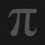# Sequence, Progression and Series

Consider a set (here, repetitions of members are allowed) of numbers that are arranged according to a specific rule. If the rule of their arrangement is logical, the set of numbers is said to be a sequence. On the other hand, if the rule of their arrangement has a mathematical formula, the set of numbers is said to be a progression.
Consider the following examples :

P = {2, 3, 5, 7, 11, . . . } ; Q = {3, 1, 4, 1, 5 , 9, 2, 6, 5, . . } ; R = {2, 4, 6, 8, 10, . . .} & S = {2, 4, 8, 16, 32, 64, . . .}

The set of prime numbers (P) and the set of numbers of π (Q) are sequences but not a progressions. The members in these sets have a logic but not a mathematical formula to be predicted. The sets R and S are progressions as well as sequences.

Every progression is a sequence but the converse is not true.

When the terms of a sequence or a progression are added or subtracted, the mathematical structure so obtained is a series.

Arithmetic Series: 5 + 9 + 13 + 17 + . . .
Geometric Series: 1/2 + 1/4 + 1/8 + . .
Special series: 1.2 + 2.3 + 3.4 + . . .
Grandi's Series: 1 - 1 + 1 - 1 + 1 - 1 + . . .Note by Shubham Poddar
2 years, 5 months ago

This discussion board is a place to discuss our Daily Challenges and the math and science related to those challenges. Explanations are more than just a solution — they should explain the steps and thinking strategies that you used to obtain the solution. Comments should further the discussion of math and science.

When posting on Brilliant:

• Use the emojis to react to an explanation, whether you're congratulating a job well done , or just really confused .
• Ask specific questions about the challenge or the steps in somebody's explanation. Well-posed questions can add a lot to the discussion, but posting "I don't understand!" doesn't help anyone.
• Try to contribute something new to the discussion, whether it is an extension, generalization or other idea related to the challenge.

MarkdownAppears as
*italics* or _italics_ italics
**bold** or __bold__ bold
- bulleted- list
• bulleted
• list
1. numbered2. list
1. numbered
2. list
Note: you must add a full line of space before and after lists for them to show up correctly
paragraph 1paragraph 2

paragraph 1

paragraph 2

[example link](https://brilliant.org)example link
> This is a quote
This is a quote
    # I indented these lines
# 4 spaces, and now they show
# up as a code block.

print "hello world"
# I indented these lines
# 4 spaces, and now they show
# up as a code block.

print "hello world"
MathAppears as
Remember to wrap math in $$ ... $$ or $ ... $ to ensure proper formatting.
2 \times 3 $2 \times 3$
2^{34} $2^{34}$
a_{i-1} $a_{i-1}$
\frac{2}{3} $\frac{2}{3}$
\sqrt{2} $\sqrt{2}$
\sum_{i=1}^3 $\sum_{i=1}^3$
\sin \theta $\sin \theta$
\boxed{123} $\boxed{123}$